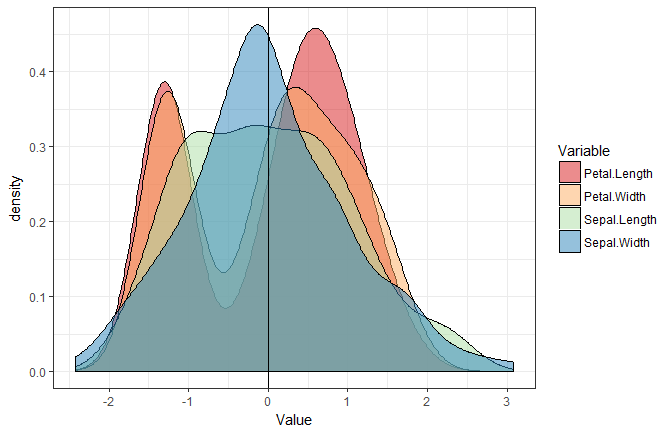Want to share your content on R-bloggers? click here if you have a blog, or here if you don't.

## Standardize / Normalize / Z-score / Scale

The `standardize()` function allows you to easily scale and center all numeric variables of a dataframe. It is similar to the base function `scale()`, but presents some advantages: it is tidyverse-friendly, data-type friendly (i.e., does not transform it into a matrix) and can handle dataframes with categorical data.

```library(psycho)
library(tidyverse)

z_iris <- iris %>%
psycho::standardize()

summary(z_iris)

Species    Sepal.Length       Sepal.Width       Petal.Length
setosa    :50   Min.   :-1.86378   Min.   :-2.4258   Min.   :-1.5623
versicolor:50   1st Qu.:-0.89767   1st Qu.:-0.5904   1st Qu.:-1.2225
virginica :50   Median :-0.05233   Median :-0.1315   Median : 0.3354
Mean   : 0.00000   Mean   : 0.0000   Mean   : 0.0000
3rd Qu.: 0.67225   3rd Qu.: 0.5567   3rd Qu.: 0.7602
Max.   : 2.48370   Max.   : 3.0805   Max.   : 1.7799
Petal.Width
Min.   :-1.4422
1st Qu.:-1.1799
Median : 0.1321
Mean   : 0.0000
3rd Qu.: 0.7880
Max.   : 1.7064
```

But beware, standardization does not change (and “normalize”) the distribution!

```z_iris %>%
dplyr::select(-Species) %>%
gather(Variable, Value) %>%
ggplot(aes(x=Value, fill=Variable)) +
geom_density(alpha=0.5) +
geom_vline(aes(xintercept=0)) +
theme_bw() +
scale_fill_brewer(palette="Spectral")
```